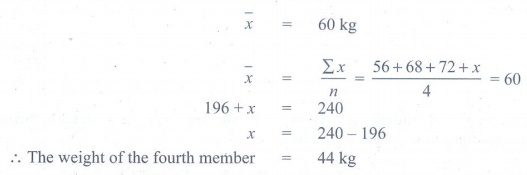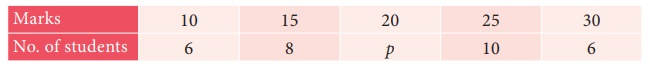Home | | Maths 9th std | Exercise 8.1: Statistics: Measures of Central Tendency, Arithmetic Mean

# Exercise 8.1: Statistics: Measures of Central Tendency, Arithmetic Mean

Maths : Statistics: Book Back, Exercise, Example Numerical Question with Answers, Solution : Exercise 8.1: Statistics: Measures of Central Tendency, Arithmetic Mean

Statistics: Measures of Central Tendency, Arithmetic Mean

Exercise 8.1

1. In a week, temperature of a certain place is measured during winter are as follows 26oC, 24oC, 28oC, 31oC, 30oC, 26oC, 24oC. Find the mean temperature of the week.

Solution:2. The mean weight of 4 members of a family is 60kg.Three of them have the weight 56kg, 68kg and 72kg respectively. Find the weight of the fourth member.

Solution:3. In a class test in mathematics, 10 students scored 75 marks, 12 students scored 60 marks, 8 students scored 40 marks and 3 students scored 30 marks. Find the mean of their score.

Solution:4. In a research laboratory scientists treated 6 mice with lung cancer using natural medicine. Ten days later, they measured the volume of the tumor in each mouse and given the results in the table.Find the mean.

Solution:5. If the mean of the following data is 20.2, then find the value of pSolution:6. In the class, weight of students is measured for the class records. Calculate mean weight of the class students using Direct method.Solution:7. Calculate the mean of the following distribution using Assumed Mean Method:Solution:8. Find the Arithmetic Mean of the following data using Step Deviation Method:Solution:Tags : Numerical Problems with Answers, Solution | Statistics | Maths , 9th Maths : UNIT 8 : Statistics
Study Material, Lecturing Notes, Assignment, Reference, Wiki description explanation, brief detail
9th Maths : UNIT 8 : Statistics : Exercise 8.1: Statistics: Measures of Central Tendency, Arithmetic Mean | Numerical Problems with Answers, Solution | Statistics | Maths